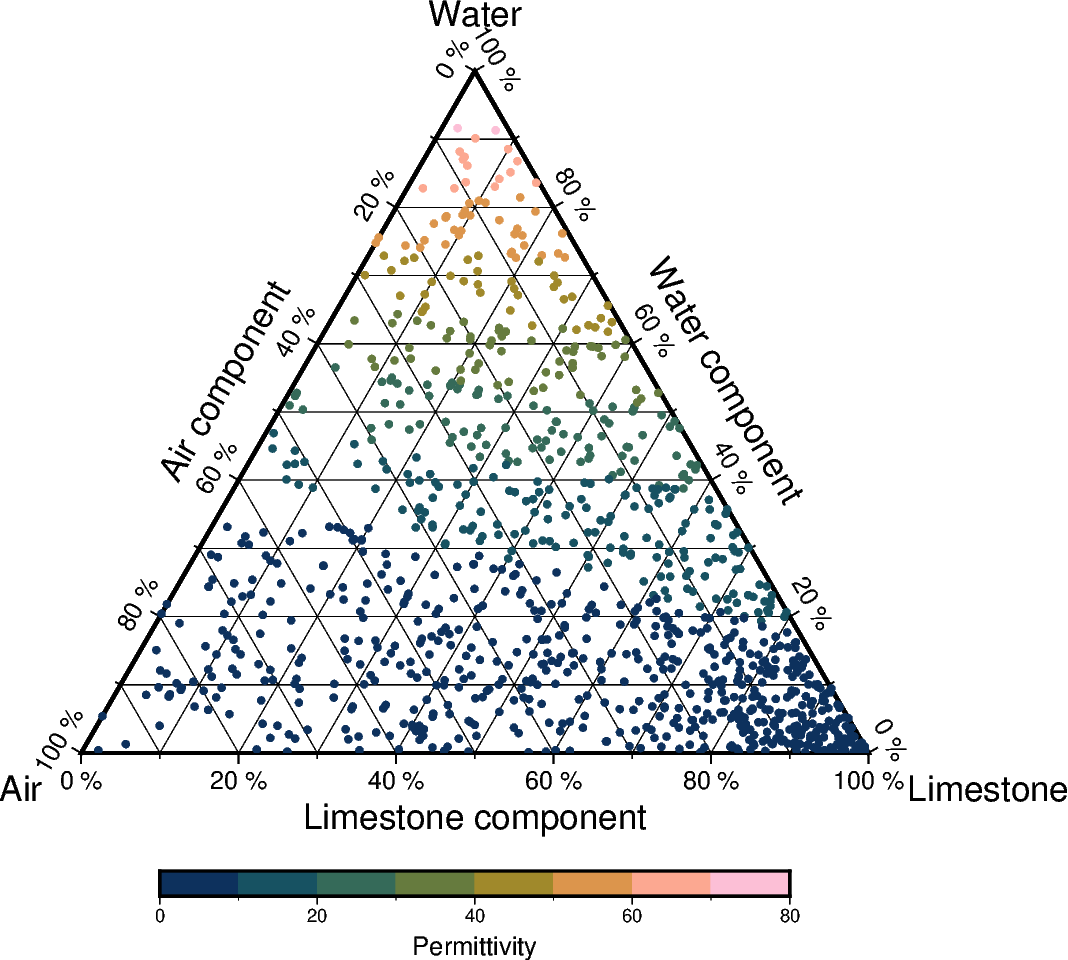# Ternary diagram

The `pygmt.Figure.ternary` method can draw ternary diagrams. The example shows how to plot circles with a diameter of 0.1 centimeters (`style="c0.1c"`) on a 10-centimeter-wide (`width="10c"`) ternary diagram at the positions listed in the first three columns of the sample dataset `rock_compositions`, with default annotations and gridline spacings, using the specified labeling defined via `alabel`, `blabel`, and `clabel`. Points are colored based on the values given in the fourth columns of the sample dataset via `cmap=True`.```import pygmt

fig = pygmt.Figure()

# Define a colormap to be used for the values given in the fourth column
# of the input dataset
pygmt.makecpt(cmap="batlow", series=[0, 80, 10])

fig.ternary(
data,
region=[0, 100, 0, 100, 0, 100],
width="10c",
style="c0.1c",
alabel="Limestone",
blabel="Water",
clabel="Air",
cmap=True,
frame=[
"aafg+lLimestone component+u %",
"bafg+lWater component+u %",
"cagf+lAir component+u %",
],
)

# Add a colorbar indicating the values given in the fourth column of
# the input dataset
fig.colorbar(position="JBC+o0c/1.5c", frame=["x+lPermittivity"])
fig.show()
```

Total running time of the script: ( 0 minutes 0.719 seconds)

Gallery generated by Sphinx-Gallery